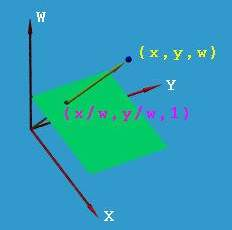Language :
 Picture : Type :[|jpg|gif|jpeg|png|] Byte :[＜2000KB] Language : 中文(简体) 中文(繁體) English 日本 한국어 Deutsch Française Ελληνικά Россию svenska Nederlandse Polska Український dansk български Italiano Icelandic român suomen kieli Galicia Türk Pilipino Català český hrvatski Latvijas Lietuvos македонски norsk språk Српски језик slovenský jazyk slovenščina Magyar nyelv فارسی Português ไทย Español Bahasa Indonesia Ngôn ngữ Việt Nam العربية Gaeilge shqiptar eesti Беларускія Die Boole-taal (Afrikaans) Malti Melayu lugha ya Kiswahili Cymraeg עברית שפה ייִדיש हिन्दी esperanto bosanski اردو زبان Azərbaycan ქართული Kreyòl ayisyen Euskal հայերեն ગુજરાતી ಕನ್ನಡ latin ພາສາລາວ বাংলা ভাষা తెలుగు தமிழ் மொழி ខ្មែរ | Check code :[Visitor (112.0.*.*)]answers [Chinese ] Time :2023-09-16Homogeneous coordinatesThe so-called homogeneous coordinate is to represent a vector that was originally n dimensions with an n 1 dimensional vector. For example, the homogeneous coordinates of a two-dimensional point (x,y) are expressed as (hx,hy,h). It can be seen that the homogeneous representation of a vector is not unique, and the homogeneous coordinates h with different values represent the same point, for example, the homogeneous coordinates (8,4,2), (4,2,1) represent two-dimensional points (4,2).So what is the need to introduce homogeneous coordinates and what are its advantages?Many graphics applications involve geometric transformations, mainly including translation, rotation, and scaling. When these transformations are calculated using matrix expressions, translation is matrix addition, rotation and scaling are matrix multiplication, which can be expressed as p' = p *m1 m2 (m1 rotation scale matrix, m2 is the translation matrix, p is the original vector, p' is the transformed vector). The purpose of introducing homogeneous coordinates is mainly to combine multiplication and addition in matrix operations, expressed in the form p' = p*M. That is, it provides an effective method for transforming a set of points in two-dimensional, three-dimensional or even high-dimensional space from one coordinate system to another by using matrix operations.Second, it can represent points at infinity. If h = 0 in homogeneous coordinates of n 1 dimensions, it actually represents an infinity point in n-dimensional space. For homogeneous coordinates (a,b,h), leave a,b unchanged,_The process of V_=(x1*x1,y1*y1,z1*z1)^1/2 represents the process by which a point in the standard coordinate system gradually moves towards infinity along the line ax by=0.